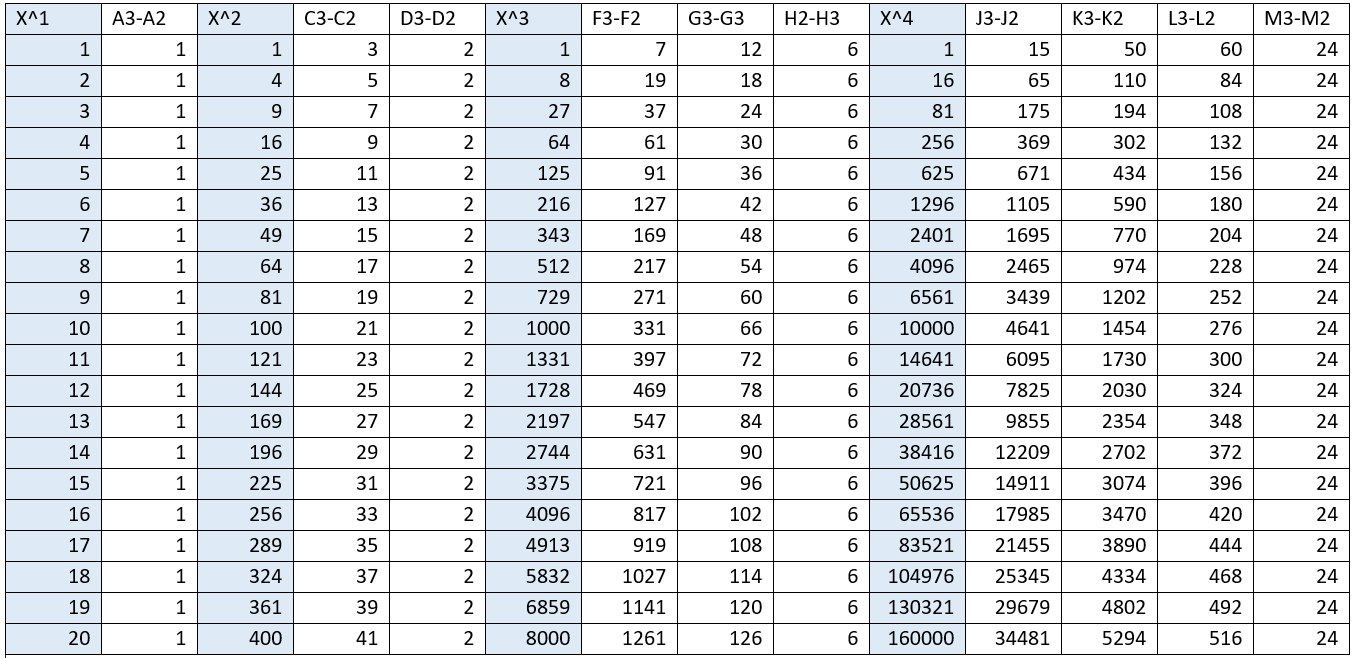# Finding a linear pattern in the powers of integers

I wondered whether I could find a pattern within in the powers of integers. At first sight, it seems like there are no linear differences between the powers. Namely, 2^1=2, 2^2,=4, 2^3=8, 2^4=16, 2^5=32, and so on (you can also look ahead at table 1 to find more examples). In 2^X, the difference between each power is 2,4,8,20 respectively. As you can see in the next picture, the different powers per integer seem to be drifting away from each other. That is no surprise, because we know that 2*2 (4) is way less than 2*2*2 (8). But is there any underlying pattern here?Differences in outcomes per exponent plotted for 1,2,3,4. And exponents ^1,^2,^3,^4. I only plotted these because the difference deviates very much quite quickly

To find a pattern, I took several integers (1-100), (depicted in the rows of table 1), and their powers X^2, X^3, X^4, X^5, and X^6 (highlighted in blue until the power of 4 in table 1). The first thing I noticed when doing this, is that the differences between the outcomes of the power, X^1 is always 1. Aka, the differences between 1,2,3,4,5,6 etc. per integer is equal to 1 (also shown in column (A3-A2) in table 1. This was a clue for me. So I took the outcomes of the power X^2 and calculated the difference, which was 3,5,7,9,11 (column C3-C2). Not the same regularity as x^1, but it was not hard to recognize that the difference from that difference was 2. I went on to X^3, took three times the difference and found the number 6 (H2-H3). I went to X^4, took 4 times the difference and found the number 24. I went too X^5, the five time difference was 120, and with X^6, the sixth time difference a constant of 720 remained.Table 1.

So I found a regularity between the integers of each power when taking the difference according to the amount of powers. I depicted the regular value’s in table 2. In table 2, the sequence seems a bit weird at first. But if you multiply each integer by the exponent of the following integer, you get the following number in the sequence. Namely, 2*3=6, 6*4=24, 24*5=120, and 120*6=720 (there are more ways to do this). So we are back at 1,2,3,4,5,6.

Conclusions
I found a regular pattern within the powers but in quite a weird way. You can find the regularity when you take the amount of differences between the powers over the integers. So with X^1, you take 1 difference (of the outcome), and with X^4 you take four differences (of the outcomes). This gives you a sequence which is regular if you multiply the integer by the power of the following integer. You’ll get 1,2,3,4,5,6 … It was fun to find this. Nevertheless, I don’t know the exact implications of this finding. I can imagine that it becomes easier to find the outcomes of a sequences (using exponents) by using this method. I’m no formal mathematician, so I didn’t put effort in making a formula using this method and applying it. This takes me too much time, given the fact that I’m uncertain about its implications.

If you have any feedback, questions, or know anything about the implications, I would love to hear from you!

Edit:
I later got the following response on “Mymathforum” after posting my blog there to get some feedback, “that the numbers 1, 2, 6, 24, 120, 720, etc., can be written as n! for the nth such number. Thus, 1! = 1, 2! = 2, 3! = 6, etc. By convention, 0! = 1. Note that n! may be read as n factorial. Factorials have lots of interesting properties.”

1.Adam

I have known about this and been examining it off and on in my spare time for years. My teachers and professors always dismissed it as non-significant. There are other interesting properties that you should try to find.

•justintimm

Hey Adam, Thanks for your Comment! It’s interesting stuff right? I have to say, I also don’t know completely what it means but I believe it is something. I once posted this blog on a math forum (to get some feedback) and there was some guy working with it and he found more patterns: https://mathforums.com/threads/finding-regularity-within-exponents.344394/

2.JulieAnn

I just spent parts of the last 3 days playing with numbers and found the same pattern. I have no formal math education besides HS, over 35 years ago. It was a struggle because I reverse numbers. I was searching online to see if I was right. Glad I found your information. Thanks.
JulieAnn

•justintimm

That is delighting to hear, I’m curious how and why you got there!

•JulieAnn

Over 25 years ago when I first read the Bible I saw patterns that no one else saw or believed were there. I tried to dismiss the patterns because of what everyone else said and believed, but the potential multitude of Bible number patterns made me more interested in the number patterns I also saw but didn’t investigate either. Reversing numbers makes it a daunting and challenging task. Patterns in numbers help check for accuracy, but are challenging to discover with number reversal.

As for the how, I just play with numbers like I do with the Bible. I think I discovered two other patterns or one double pattern within the pattern that we found.

As for professors who say the patterns don’t mean anything, every pattern of which one does not know how to utilize may appear useless or nothing. Those who think they have the sum of knowledge will never learn more.